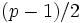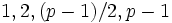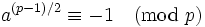# Quadratic nonresidue that is not minus one is primitive root for safe prime

## Statement

Supposeis a safe prime, i.e.,is an odd prime such thatis also prime (in other wordsis a Sophie Germain prime). Then, ifis a quadratic nonresidue modulothat is not congruent tomodulo, thenis a primitive root modulo.

## Proof

Given: A safe prime, a quadratic nonresiduemoduloother than.

To prove:is a primitive root modulo.

Proof: By Fermat's little theorem, the order ofmodulois a factor of. The only factors ofare. (If, two of these factors become equal).

• The order ofcannot beorsinceby fact (1).
• The order ofcannot besince the only element of order two is, andis notmoduloby assumption.

This forces the order to be, sois a primitive root.